Courses

# Simplex Method And Transportation Model - MCQ Test 1

## 15 Questions MCQ Test RRB JE for Mechanical Engineering | Simplex Method And Transportation Model - MCQ Test 1

Description
This mock test of Simplex Method And Transportation Model - MCQ Test 1 for Mechanical Engineering helps you for every Mechanical Engineering entrance exam. This contains 15 Multiple Choice Questions for Mechanical Engineering Simplex Method And Transportation Model - MCQ Test 1 (mcq) to study with solutions a complete question bank. The solved questions answers in this Simplex Method And Transportation Model - MCQ Test 1 quiz give you a good mix of easy questions and tough questions. Mechanical Engineering students definitely take this Simplex Method And Transportation Model - MCQ Test 1 exercise for a better result in the exam. You can find other Simplex Method And Transportation Model - MCQ Test 1 extra questions, long questions & short questions for Mechanical Engineering on EduRev as well by searching above.
QUESTION: 1

### Simplex method of solving linear programming problem uses

Solution:

Simplex method of solving linear programming problem uses only the corner points of the feasible region.

QUESTION: 2

Solution:
QUESTION: 3

### Which one of the following is true in case of simplex method of linear programming?

Solution:
QUESTION: 4

The supply at three sources is 50, 40 and 60 units respectively whilst the demand at the four destinations is 20, 30, 10 and 50 units. In solving this transportation problem

Solution:
QUESTION: 5

Consider the Linear Programme (LP)
Maximize 4x + 6y
subject to 3x + 2y ≤  6
2x + 3y ≤ 6
x, y ≥ 0

Q.

After introducing slack variables s and t, the initial basic feasible solution is represented by the tableau below (basic variables are s = 6 and t = 6, and the objective function value is 0).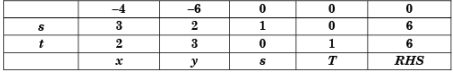After some simplex iteration, the following tableau is obtained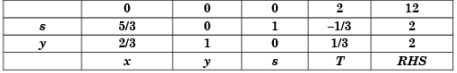From this, one can conclude that

Solution:

As Cj = 0, 0 for x and y respectively therefore It is an optimal solution but not unique.

QUESTION: 6

Consider the Linear Programme (LP)
Maximize 4x + 6y
subject to 3x + 2y ≤  6
2x + 3y ≤ 6
x, y ≥ 0

Q.

The dual for the LP in Q 2 is:

Solution:

Duplex method:
Step-I: Convert the problem to maximization form so Choice may be (b) or (c).
Step-II: Convert (≥) type con straints if any to (≤) type by multiplying such constraints by (–1) so our choice is (b).

QUESTION: 7

A company has two factories S1, S2 and two warehouses D1, D2. The supplies from S1 and S2 are 50 and 40 units respectively. Warehouse D1 requires a minimum of 20 units and a maximum of 40 units. Warehouse D2 requires a minimum of 20 units and, over and above, it can take as much as can be supplied. A balanced transportation problem is to be formulated for the above situation. The number of supply points, the number of demand points, and the total supply (or total demand) in the balanced transportation problem respectively are:

Solution:
QUESTION: 8

Which one of the following subroutines does a computer implementation of the simplex routine require?

Solution:
QUESTION: 9

A firm is required to procure three items (P, Q, and R). The prices quoted for these items (in Rs.) by suppliers S1, S2 and S3 are given in table. The management policy requires that each item has to be supplied by only one supplier and one supplier supply only one item. The minimum total cost (in Rs.) of procurement to the firm is: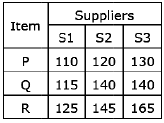Solution:
QUESTION: 10

In the solution of linear programming problems by Simplex method, for deciding the leaving variable

Solution:
QUESTION: 11

In order for a transportation matrix which has six rows and four columns, not to be degenerate, how much must be the number of allocated cells in the matrix?

Solution:

m + n - 1 = 4 + 6 - 1 = 9

QUESTION: 12

A tie for leaving variables in simplex procedure implies:

Solution:
QUESTION: 13

Consider the following statements:

The assignment problem is seen to be the special case of the transportation problem in which 1. m = n
2. All ai =  1
3. xij = 1
(The symbols have usual meaning)

Which of the statements given above are correct?

Solution:

3 - is wrongQUESTION: 14

Match List-I (Persons with whom the models are associated) with List-II (Models) and select the correct answer: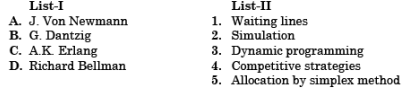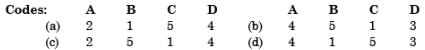Solution:
QUESTION: 15

Which one of the following is not the solution method of transportation problems?

Solution:

Hungarian method is used in assignment problem (but remember assignment problem is a special case of transportation problem).